Multiple Linear Regression in Python: With an Example

By: Ram on Jun 18, 2020

When people buy a house, the price of a house is an important consideration for the purchase decision but definitely not the only one. Setting up right price helps in closing the deal faster. In this example, we are building a regression model to predict house price based on attributes of a house.

Important reasons we selected multiple regression technique to build house price estimation model are:

• Decision variable – price of house is continuous variable
• Easy to understand and explain: Easy to find important features and their role in estimating house price
• Easy deployment: once a house price prediction model is finalized, the model has a simple equation with basic mathematical operations only, so can be implemented in any technology set up without need of installing analytics tools or library.

Drivers of House Price

In an enterprise project, we spend significant time to understand business context for any Data Science project. In this house price estimation, we need to identify drivers of house prices, find data sources and extract from all the data sources possible to ensure the model is not ignoring an important feature/s.

Some of the key dimensions influencing house prices are:

• House: Size, layout, Number of rooms, construction year etc
• Locality:  Locality, area, distance to hospital, malls, railway station and other facilities etc
• Builder: Reputation of a builder, years of operations, number of such properties built etc
• Economic: Interest Rate and Other Economic variables drive house demands

What additional factors or dimension can impact house prices?

For the current example, we have only limited set of variables available for us to build the model. We will explore that dataset in the next section.

Data Exploration

For the current scenario, we have MEDV - house price as a decision or dependent variable and a list of independent variables. The list of variables and their descriptions are as follow.

Data Sample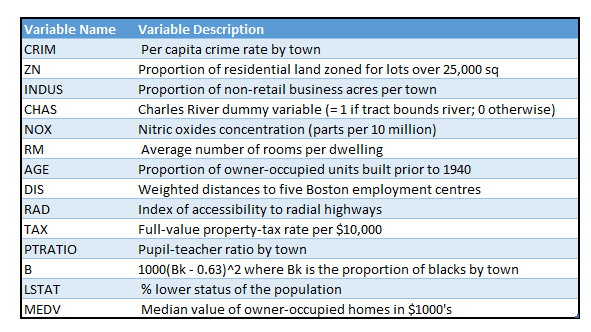We will use some of the Python libraries for the reading and exploring data and then building the regression model.

import pandas as pd

Before building a model, we need to explore the data. Some of the key steps in data exploration are:

• Summary statistics: Check for missing values and outliers for each of the variables
• Bivariate Analysis: Correlation analysis to understand the relationship between house price and each of the house attributes.
• Feature Engineering: Creating new features based on categorical variables, transforming continuous variables, adding new variables using business logic and context, etc

Summary Statistics

# Summary Statistics

house.describe()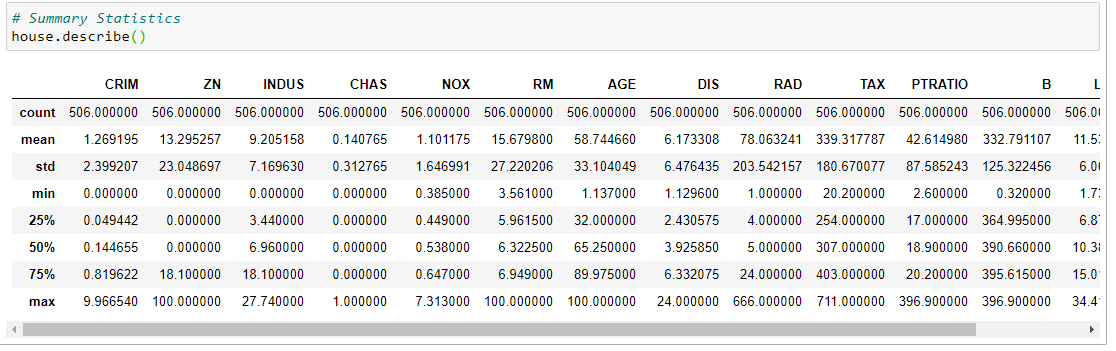Missing Values

# Check if missing values

def missing_values_table(df):

mis_val = df.isnull().sum()

mis_val_percent = 100 * df.isnull().sum() / len(df)

mis_val_table = pd.concat([mis_val, mis_val_percent], axis=1)

mis_val_table_ren_columns = mis_val_table.rename(

columns = {0 : 'Missing Values', 1 : '% of Total Values'})

mis_val_table_ren_columns = mis_val_table_ren_columns[

mis_val_table_ren_columns.iloc[:,1] != 0].sort_values(

'% of Total Values', ascending=False).round(1)

print ("Your selected dataframe has " + str(df.shape) + " columns.\n"

"There are " + str(mis_val_table_ren_columns.shape) +

" columns that have missing values.")

return mis_val_table_ren_columns

# source: https://stackoverflow.com/questions/26266362/how-to-count-the-nan-values-in-a-column-in-pandas-dataframe

missing_values_table(house)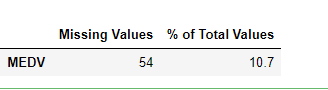For this data sample, 54 records have missing house price values. Typically, we investigate the reasons of missing values and then decide action on missing value treatment. There are multiple approaches for missing value treatment, for simplicity, we are removing these rows/observations from further steps.

dropna() method helps us in dropping the rows with missing values.

# missing count

print(house['MEDV'].isna().sum())

# Removing missing values

house.dropna(inplace=True)

print('missing values after drop',house['MEDV'].isna().sum())

Outlier Checks

For checking outliers, there are a few methods available such as quantile, box plot and histogram.

A comparison between mean and median can also be very useful.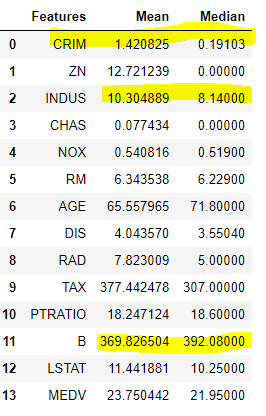Based on Mean and Median comparisons, 3 variables seem to have outliers. Box Plot can also be used for exploring variables for outliers. Now we can further explore these features.

#house.boxplot(['CRIM'])

house.boxplot(['INDUS'])

#house.boxplot(['B'])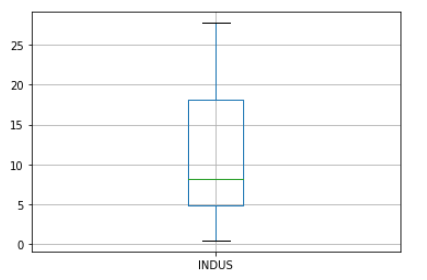We can do the capping of values to manage outliers. But in this example, the outlier values are not at very critical level, so let’s not do anything for now.

Bivariate Analysis

We should also explore bivariate relationship between each of the independent variables and the target variable. Scatter Plot and Correction Plot are the good tools for us to use.

# Scatter Plot
import matplotlib.pyplot as plt
plt.scatter(house['CRIM'], house['MEDV'])
plt.title('Median House Price Vs Crime Rate')
plt.xlabel('Crime Rate')
plt.ylabel('House Price')
plt.show()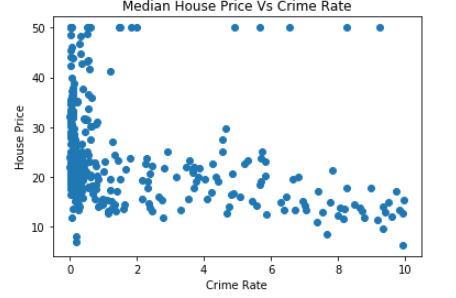Not very clear but seems to have a negative relationship – when crime rate is higher, the median house price is lower.

Considering most of the independent variables are continuous and so is the target variable, so we can also find correlation between each of the combinations.

# Correlation Plot

import seaborn as sns

corr = house.corr()

ax = sns.heatmap(

corr,

vmin=-1, vmax=1, center=0,

cmap=sns.diverging_palette(20, 220, n=200),

square=True

)

ax.set_xticklabels(

ax.get_xticklabels(),

rotation=45,

horizontalalignment='right'

);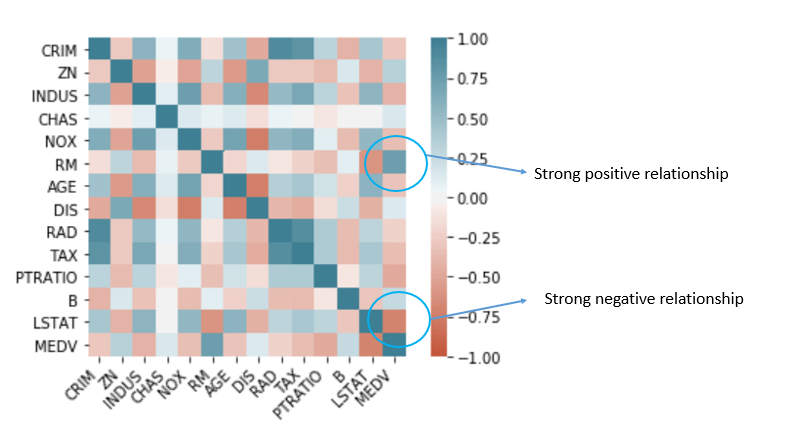We should look at the combinations which have a high positive or negative correlation coefficient. Also, the focus should be given to the correlation of Median House Prices (MEDV) with each of the independent variables as MEDV – House Price which is the target variable in this example.

Note that variable RM has a strong positive correlation and LSTAT has a strong negative correlation with target variables.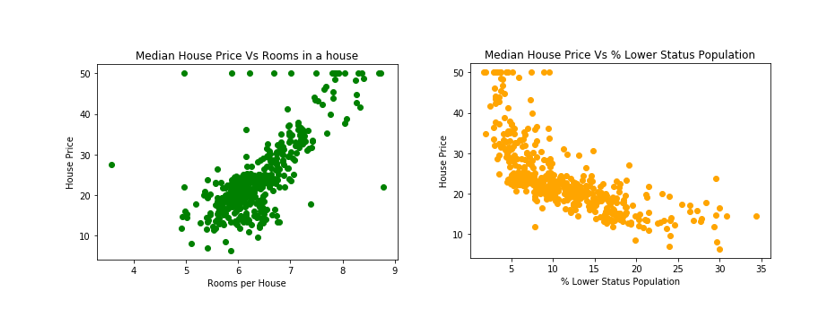Feature Engineering: Variable Transformations

Variable transformation is one of the steps in Feature engineering to find a better model.  5 common variable transformations are Inverse (1/x), Square Root, Square (x2), Log(x) and Exp(x)/ex.

To make things easy, we can write a function for these 5 transformations and they can apply on the list of input columns.

# Feature Engineering
import pandas as pd
import numpy as np

# Log
house['log_LSTAT'] = np.log(house['LSTAT']+1+np.abs(np.min(house['LSTAT'])))
# Exponential
house['exp_LSTAT'] = np.exp(house['LSTAT']/np.max(house['LSTAT']))
# Square
house['sqr_LSTAT'] = np.power(house['LSTAT'],2)
#Square Root
house['sqrt_LSTAT'] = np.sqrt(house['LSTAT']+1+np.abs(np.min(house['LSTAT'])))
# Inverse
house['inv_LSTAT'] = 1/(house['LSTAT']+1+np.abs(np.min(house['LSTAT'])))

To keep some basic things in perspective, we have written in slightly complicated transformation steps as we know that the logarithm is not valid for negative & zero values and similarly square root is not a real number for negative values.
Now, you can do a similar transformation for other independent variables.
If we had a list of categorical features, we would have required to create dummy variables for the features set.
Now, the sample is ready for Machine Learning models. We will split the sample to a Training (or Development) sample and Test (or Validation) sample.

Train and Test Samples

We can create Train/Development and Test/Validation samples using Python function. We can split randomly and in the ratio of 70:30, 60:40, and 50:50.

Before that, we can split features and house price data into two separate data frames.

#Features and Label Data

house_features = house.drop('MEDV', axis=1)

house_prices = house['MEDV']

We have chosen 60% as development and 40% as validation samples. This can be done using train_test_split() function as follow.

# Split the data into Dev/Train and Val/Test

from sklearn.model_selection import train_test_split

house_features_dev,house_features_val,house_prices_dev,house_prices_val=train_test_split(house_features,house_prices,test_size=0.4,random_state=0)

Now, we can train our regression model on the training sample.

Regression Model Development

In the regression model, we need to identify variables that are good predictors. We can identify the combination of features which give the best R2 and Adjusted R2 is the final model. If the features are more, it can be a daunting task to find that combination. There are a number of approaches are available for variable selection.
We will try one the simplest approach, selecting the variable based on correlation coefficient.  We have already plotted correction matrix and RM and LSTAT have strong relationship with the target variable: MEDV- house price.
For simple linear regression, we can try with one variable and let’s take LSTAT feature for simple regression model development.
For simple regression, the Model Equation with MEDV as target variable and LSTAT as independent variable or feature will be:
MEDV = B0 + B1* LSTAT
Based on the sample of data points, we need to find the value of B0 and B1. We are using statsmodels for building Regression Model but it does not estimate B0 by default. So, we need to use add_constant() function to ask statsmodels to estimate B0.
# Simple Linear Regression
import statsmodels.api as sm
simpleOLS.summary()

Model Output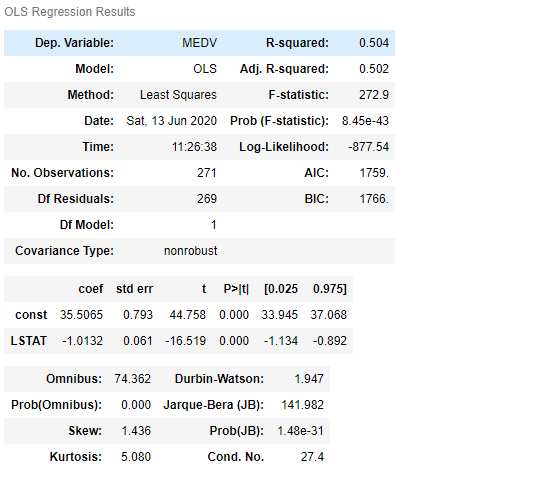Model Output Interpretation
Now the model output has B0 and B1. The value of B0 is 35.5065 and B1 is -1.0132.  The negative sign of B1 suggests that when the value of LSTAT is going up, the value of house prices will go down. And, the change in house price for per unit change in CSTAT is B1 value. The below graph helps you to visualize the concept of B0 and B1.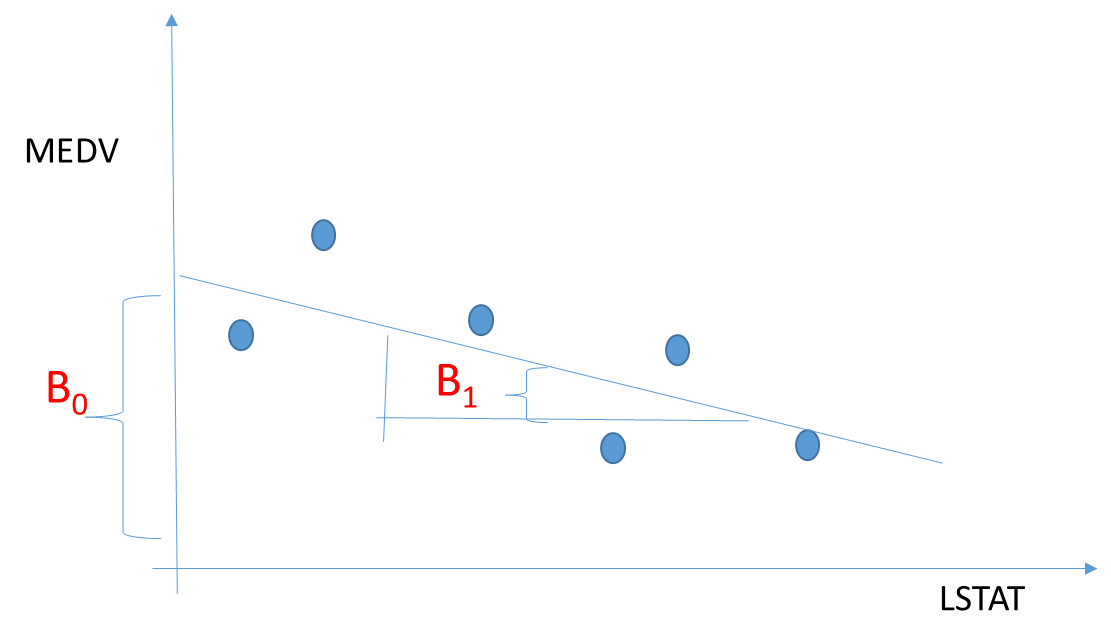P-Value indicates the significance of the LSTAT in predicting value house prices. If P-Value is less than 0.01, we can keep the variable in the model for 1% significance.

R2 gives an indication of the predictive power or accuracy level of the model. It is % of variance explained by a set of feature/s. The below graph explains the concept of variance and calculation of R2.

In this example,  50% variance in MEDV is explained by LSTAT feature.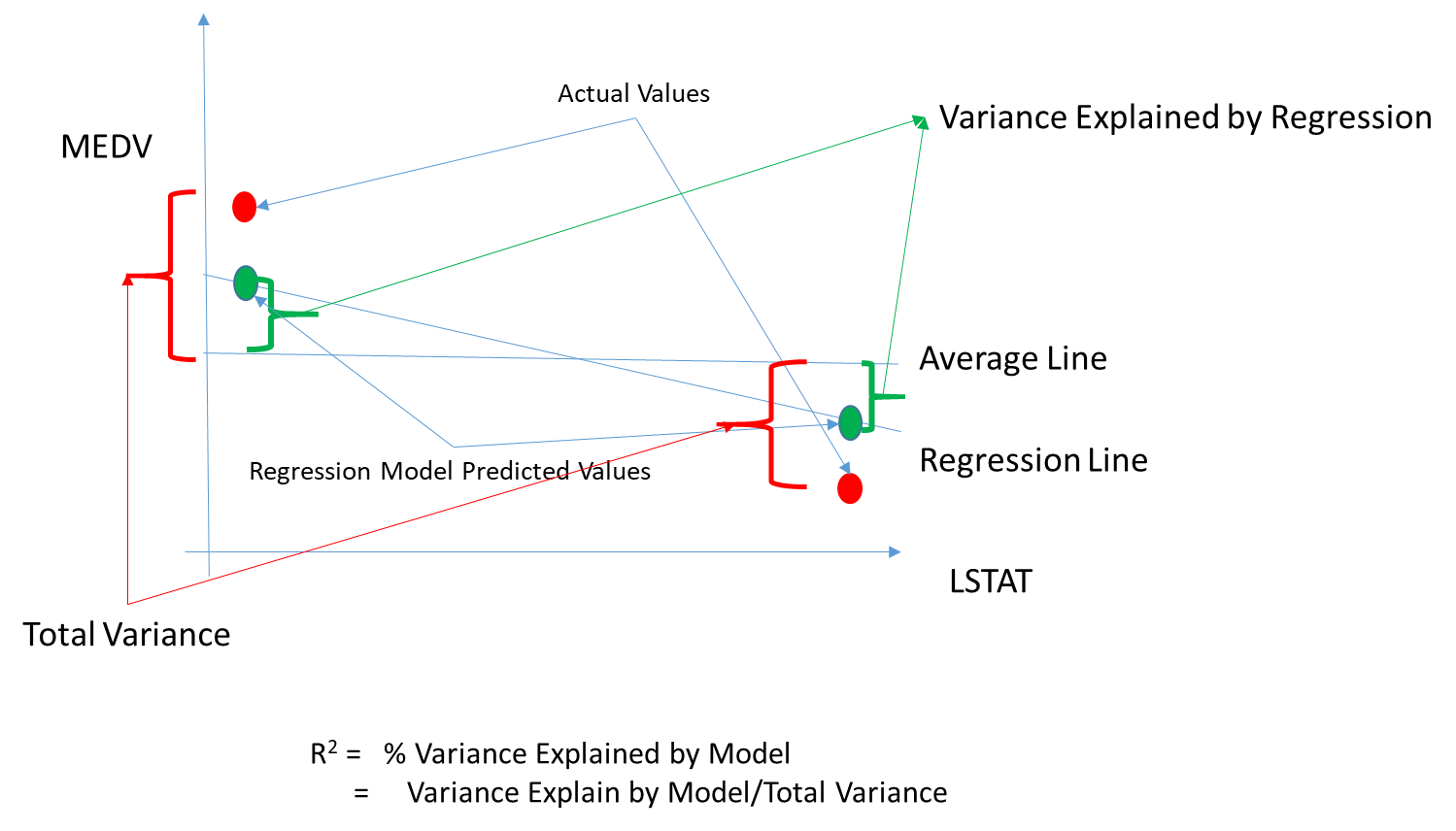Adjusted R2 calculation penalizes a model with more features as R2 values only increase with more features/variables.

Now, we are building a multiple regression model using all the available features.

When we are selecting a model, we need all the features to be significant and R2/Adj R2 is high.

Multiple Regression Model

# Updated Feature list

simpleOLS.summary()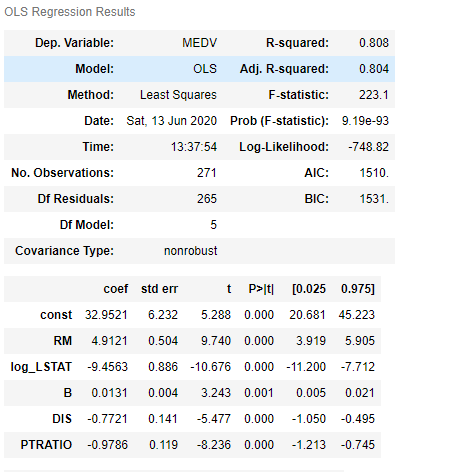Model Output Interpretation

The model has 5 features and all of these features are significant - very low P-value so good to keep in the model.

R2 is 0.80 which means that 80% of the variance is explained by the model. So, it is a good model. Further steps can be explored to improve the accuracy of the model.

Now, we need to use the model and score (estimate house price) the training sample. Compare the estimated house price vs actual house prices. Also, compare the model statistics on Training and Test Data samples to see if there is any significant drop in the model performance.

Scoring Validation Sample and Validate the Model

Using the model developed, we score the test sample and compare the predicted and actual house prices.

# Score or Predict House price: Test Sample

# Compare Predicted and Actual House Prices in Validation/Testing Sample

# Scatter Plot

import matplotlib.pyplot as plt

plt.scatter(house_prices_val, pred_house_price_val)

plt.title('Test Sample: Predicted Vs Actual')

plt.xlabel('Actual House Price')

plt.ylabel('Predicted House Price')

plt.show()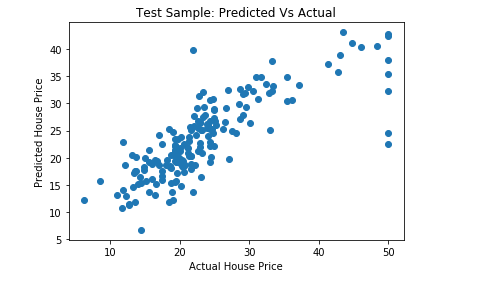Tags
Most PopularJun 18, 2020Jul 23, 2020Jun 19, 2020Jun 19, 2021